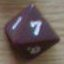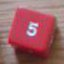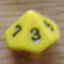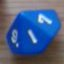Need help with a slightly complicated dice function

## Need help with a slightly complicated dice function

 Fri Nov 16 00:33:33 2018   by   vxi ...It works with one d8 and one d4, but I want it to also work with multiple...- With "f:=f-max{f}+d4" I tried to remove the highest value in {f} from {f} and replace it with a new d4, but it expects a singleton (lines 8, 11, 12).How do I remove the highest value from a union and replace it with a new die roll?- With "a:=a-min{{a > min{f}}}" I tried to remove from {a} the lowest value in {a} that is higher than the lowest value in {f} (line 11).How do I do that?Here's what I have so far:1:      a:=d8;2:      f:=d4;3:      x:=0;4:      call fuel(a,f,x)5:      function fuel(a,f,x) =6:          if 4 = max{f} then7:          x:=x+4;8:          f:=f-max{f}+d4;9:          call fuel(a,f,x)10:            else if max{a} > min{f} then11:            a:=a-min{{a > min{f}}};12:            f:=f-min{f}+d4;13:            call fuel(a,f,x)14:                else x:=x+sum{f};15:                xThank you. Mon Nov 19 09:35:29 2018   by   Torben The symbol "-" is used for subtraction of numbers.  What you want is removing a number from a collection.  For that you need the "--" operator.Similarly, "+" is for addition of numbers.  To add an element to a collection, you need "U" (union).So the line `f:=f-min{f}+d4;` should instead be `f:= (f -- min f) U d4`.  The parentheses are needed because U binds stronger than --.As you can see, you don't need the curly braces when you apply min or max.I'm not sure what you want to accomplish with the line `a:=a-min{{a > min{f}}};`.  `a > min f` will return either the minimum value of f (if this is less than a) or the empty collection.  Taking the minimum of the empty collection will give a run-time error. Fri Nov 23 04:28:32 2018   by   vxi Awesome, thank you. The "--" and "U" were exactly what I needed.What I wanted to write with "{a > min{f}}" is.."the collection of numbers in {a} that are greater than the smallest number in {f}"So if {a} is {1,2,3,4} and {f} is {2,3}, that would be {3,4}.Then I want to remove the lowest one of those values from {a}. That would make {a} = {1,2,4}.All I need now is some function that can give me "the collection of numbers in {a} that are greater than the smallest number in {f}"How would one do that?